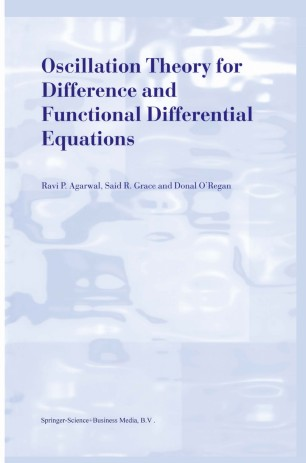# Oscillation Theory for Difference and Functional Differential Equations

• Ravi P. Agarwal
• Said R. Grace
• Donal O’ReganBook

1. Front Matter
Pages i-vii
2. Ravi P. Agarwal, Said R. Grace, Donal O’Regan
Pages 1-165
3. Ravi P. Agarwal, Said R. Grace, Donal O’Regan
Pages 166-317
4. Back Matter
Pages 318-337

### Introduction

This monograph is devoted to a rapidly developing area of research of the qualitative theory of difference and functional differential equations. In fact, in the last 25 years Oscillation Theory of difference and functional differential equations has attracted many researchers. This has resulted in hundreds of research papers in every major mathematical journal, and several books. In the first chapter of this monograph, we address oscillation of solutions to difference equations of various types. Here we also offer several new fundamental concepts such as oscillation around a point, oscillation around a sequence, regular oscillation, periodic oscillation, point-wise oscillation of several orthogonal polynomials, global oscillation of sequences of real­ valued functions, oscillation in ordered sets, (!, R, ~)-oscillate, oscillation in linear spaces, oscillation in Archimedean spaces, and oscillation across a family. These concepts are explained through examples and supported by interesting results. In the second chapter we present recent results pertaining to the oscil­ lation of n-th order functional differential equations with deviating argu­ ments, and functional differential equations of neutral type. We mainly deal with integral criteria for oscillation. While several results of this chapter were originally formulated for more complicated and/or more general differ­ ential equations, we discuss here a simplified version to elucidate the main ideas of the oscillation theory of functional differential equations. Further, from a large number of theorems presented in this chapter we have selected the proofs of only those results which we thought would best illustrate the various strategies and ideas involved.

### Keywords

Scala derivative difference equation differential equation equation function functional functional equation functions integral orthogonal polynomials proof review types variable

#### Authors and affiliations

• Ravi P. Agarwal
• 1
• Said R. Grace
• 2
• Donal O’Regan
• 3
1. 1.Department of MathematicsNational University of SingaporeSingapore
2. 2.Department of Engineering MathematicsCairo UniversityOrman, GizaEgypt
3. 3.Department of MathematicsNational University of IrelandGalwayIreland

### Bibliographic information

• DOI https://doi.org/10.1007/978-94-015-9401-1